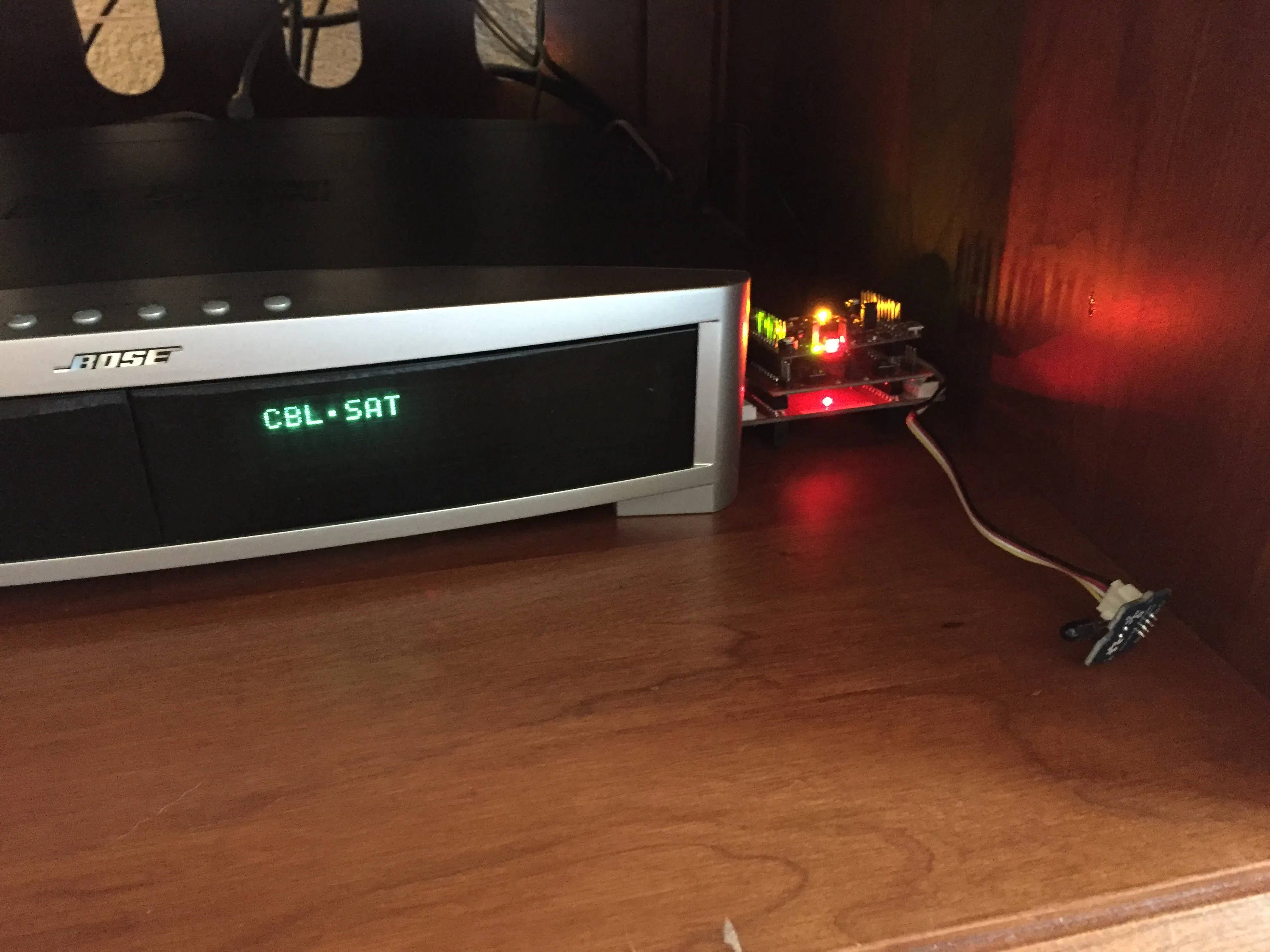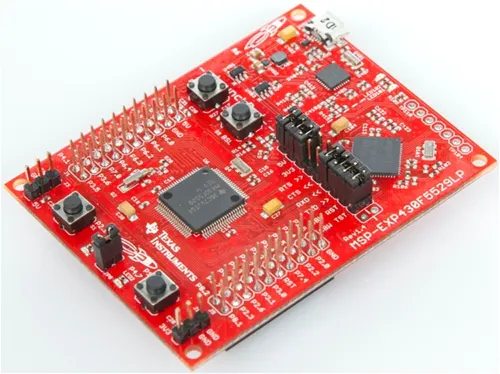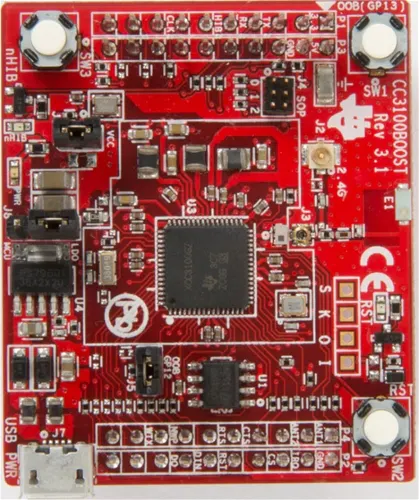# Making Old Things IoT - IR Control

This project brings a pre-IoT audio receiver up to speed using Blynk and a TI Launchpad to send IR signals through WiFi from an iPhone.

IntermediateShowcase (no instructions)4,069## Things used in this project

### Hardware components×1Texas Instruments CC3100BOOST SimpleLink CC3100 Wi-Fi BoosterPack
×1
 Seeed Studio grove IR module
×1

### Software apps and online servicesBlynkTexas Instruments Energia

## Code

### smartBose

C/C++
```#include <IRSendRev.h>
#define BIT_LEN         0
#define BIT_START_H     1
#define BIT_START_L     2
#define BIT_DATA_H      3
#define BIT_DATA_L      4
#define BIT_DATA_LEN    5
#define BIT_DATA        6
#define commandMax      4
const int ir_freq = 38;                 // 38k
IRSendRev IR;

#define BLYNK_PRINT Serial    // Comment this out to disable prints and save space
#include <SPI.h>
#include <WiFi.h>
#include <BlynkSimpleTI_CC3200_LaunchXL.h>

// You should get Auth Token in the Blynk App.
// Go to the Project Settings (nut icon).
char auth[] = "XXXXXXX";

char ssid[] = "XXXXX";
char pass[] = "XXXXX";        // Set to "" for open networks

struct cmd_t{
unsigned char dta;
} IRout[commandMax];

void setup()
{
dtaInit();
IR.setSend(40);
pinMode(pb1,INPUT_PULLUP);
Serial.begin(9600);
Blynk.begin(auth, ssid, pass);
}
int i =0;
void loop()
{
Blynk.run();
//Serial.print("Sending IRout ");
//Serial.println(i);
IR.Send(IRout[i++].dta, 38);
}
if(i>3){
i=0;
}
}
delay(100);
}

void dtaInit()
{
//Power
IRout.dta[BIT_LEN]        = 9;			// all data that needs to be sent
IRout.dta[BIT_START_H]    = 180;			// the logic high duration of "Start"
IRout.dta[BIT_START_L]    = 91;			// the logic low duration of "Start"
IRout.dta[BIT_DATA_H]     = 11;			// the logic "long" duration in the communication
IRout.dta[BIT_DATA_L]     = 33;			// the logic "short" duration in the communication
IRout.dta[BIT_DATA_LEN]   = 4;			// Number of data which will sent. If the number is other, you should increase or reduce dtaSend[BIT_DATA+x].
IRout.dta[BIT_DATA+0]     = 93;			// data that will sent
IRout.dta[BIT_DATA+1]     = 210;
IRout.dta[BIT_DATA+2]     = 50;
IRout.dta[BIT_DATA+3]     = 205;
//Set Input
IRout.dta[BIT_LEN]        = 9;			// all data that needs to be sent
IRout.dta[BIT_START_H]    = 180;			// the logic high duration of "Start"
IRout.dta[BIT_START_L]    = 91;			// the logic low duration of "Start"
IRout.dta[BIT_DATA_H]     = 11;			// the logic "long" duration in the communication
IRout.dta[BIT_DATA_L]     = 33;			// the logic "short" duration in the communication
IRout.dta[BIT_DATA_LEN]   = 4;			// Number of data which will sent. If the number is other, you should increase or reduce dtaSend[BIT_DATA+x].
IRout.dta[BIT_DATA+0]     = 93;			// data that will sent
IRout.dta[BIT_DATA+1]     = 210;
IRout.dta[BIT_DATA+2]     = 168;
IRout.dta[BIT_DATA+3]     = 87;
//Voluem Up
IRout.dta[BIT_LEN]        = 9;			// all data that needs to be sent
IRout.dta[BIT_START_H]    = 180;			// the logic high duration of "Start"
IRout.dta[BIT_START_L]    = 91;			// the logic low duration of "Start"
IRout.dta[BIT_DATA_H]     = 11;			// the logic "long" duration in the communication
IRout.dta[BIT_DATA_L]     = 33;			// the logic "short" duration in the communication
IRout.dta[BIT_DATA_LEN]   = 4;			// Number of data which will sent. If the number is other, you should increase or reduce dtaSend[BIT_DATA+x].
IRout.dta[BIT_DATA+0]     = 93;			// data that will sent
IRout.dta[BIT_DATA+1]     = 210;
IRout.dta[BIT_DATA+2]     = 192;
IRout.dta[BIT_DATA+3]     = 63;
//Volume Down
IRout.dta[BIT_LEN]        = 9;			// all data that needs to be sent
IRout.dta[BIT_START_H]    = 180;			// the logic high duration of "Start"
IRout.dta[BIT_START_L]    = 91;			// the logic low duration of "Start"
IRout.dta[BIT_DATA_H]     = 11;			// the logic "long" duration in the communication
IRout.dta[BIT_DATA_L]     = 33;			// the logic "short" duration in the communication
IRout.dta[BIT_DATA_LEN]   = 4;			// Number of data which will sent. If the number is other, you should increase or reduce dtaSend[BIT_DATA+x].
IRout.dta[BIT_DATA+0]     = 93;			// data that will sent
IRout.dta[BIT_DATA+1]     = 210;
IRout.dta[BIT_DATA+2]     = 64;
IRout.dta[BIT_DATA+3]     = 191;
}
```

### IRGrove

C/C++
Seeed's arduino Library for the IR emitter modified for the TI Launchpad
`No preview (download only).`

### BlynkLib

C/C++
Modified Blynk library to work with CC3100 BoosterPack (small, debugging changes)
`No preview (download only).`

C/C++
Used to receive an IR signal and print the results to the serial monitor. Helpful for trying to figure out codes to emit
```#include <IRSendRev.h>

#define BIT_LEN         0
#define BIT_START_H     1
#define BIT_START_L     2
#define BIT_DATA_H      3
#define BIT_DATA_L      4
#define BIT_DATA_LEN    5
#define BIT_DATA        6
#define LED GREEN_LED
const int pinRecv = 2;              // ir receiver connect to D2
IRSendRev IR;
boolean state = 0;
void setup()
{
Serial.begin(115200);
IR.Init(5);
Serial.println("init over");
pinMode(LED,OUTPUT);

digitalWrite(LED,LOW);
}

unsigned char dta;

void loop()
{
if(IR.IsDta())                  // get IR data
{
IR.Recv(dta);               // receive data to dta

Serial.println("+------------------------------------------------------+");
Serial.print("LEN = ");
Serial.println(dta[BIT_LEN]);
Serial.print("START_H: ");
Serial.print(dta[BIT_START_H]);
Serial.print("\tSTART_L: ");
Serial.println(dta[BIT_START_L]);

Serial.print("DATA_H: ");
Serial.print(dta[BIT_DATA_H]);
Serial.print("\tDATA_L: ");
Serial.println(dta[BIT_DATA_L]);

Serial.print("\r\nDATA_LEN = ");
Serial.println(dta[BIT_DATA_LEN]);

Serial.print("DATA: ");
for(int i=0; i<dta[BIT_DATA_LEN]; i++)
{
Serial.print("0x");
Serial.print(dta[i+BIT_DATA], HEX);
Serial.print("\t");
}
Serial.println();

Serial.print("DATA: ");
for(int i=0; i<dta[BIT_DATA_LEN]; i++)
{
Serial.print(dta[i+BIT_DATA], DEC);
Serial.print("\t");
}
Serial.println();
Serial.println("+------------------------------------------------------+\r\n\r\n");
}
if(dta[BIT_DATA] == 100){
state = !state;
digitalWrite(LED,state);
}
dta[BIT_DATA] = 0;
}
```

## Credits

### Chris Roberts

1 project • 20 followers
I am an applications engineer with Texas Instruments working with the Launchpad by trade, and a maker by passion!